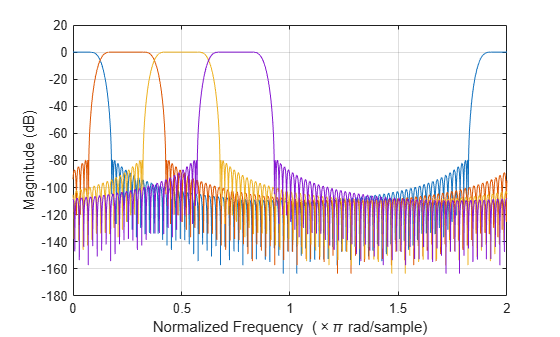# getFilters

Return matrix of channelizer FIR filters

## Syntax

``B = getFilters(obj)``
``B = getFilters(obj,ind)``

## Description

example

````B = getFilters(obj)` returns a matrix `B` of filter coefficients corresponding to each filter in the `dsp.Channelizer` System object™ filter bank. Each row contains the coefficients for the corresponding bandpass filter. The channelizer does not actually use all these filters in the implementation. It only uses the prototype lowpass filter (the first row of matrix `B`) and an FFT to implement the filter bank. The combination of polyphase implementation of the prototype lowpass and the FFT effectively implements all the filters in `B`, but does so in a very efficient manner.```

example

````B = getFilters(obj,ind)` returns the filters with indices corresponding to the elements in the vector `ind`. `ind` is a row vector of indices between `1` and `obj.NumFrequencyBands`. By default, this vector is [1:N], where N is the number of frequency bands specified in the `obj.NumFrequencyBands` property.```

## Examples

collapse all

Using the `getFilters` function, you can access the coefficients of the lowpass prototype filter and the modulated bandpass filters of the channelizer.

```channelizer = dsp.Channelizer; B = getFilters(channelizer);```

The first row corresponds to the coefficients of the prototype filter. The subsequent rows correspond to the coefficients of the respective modulated filters. Compare the first row with the coefficients returned by the `tf` function.

```b = tf(channelizer); isequal(b,B(1,:))```
```ans = logical 1 ```

The output of the `tf` function and the first row of the `B` matrix are equal.

Visualize the frequency response of the first 4 filters of the channelizer.

`fvtool(B(1,:),1, B(2,:),1, B(3,:),1, B(4,:),1);`## Input Arguments

collapse all

Input filter, specified as `dsp.Channelizer` System object. The `getFilters` function returns a matrix of filter coefficients corresponding to each filter in the channelizer filter bank.

Filter indices, specified as a row vector in the range [1 `obj.NumFrequencyBands`]. If not specified, `ind` is 1:N, where N is the number of frequency bands specified through the `obj.NumFrequencyBands` property.

Example: getFilters(channelizer,[1:4]);

Data Types: `single` | `double` | `int8` | `int16` | `int32` | `int64` | `uint8` | `uint16` | `uint32` | `uint64`

## Output Arguments

collapse all

Matrix of channelizer finite impulse response (FIR) filter coefficients, returned as a matrix. Each row in the matrix corresponds to a filter in the filter bank. The first row corresponds to the prototype filter as returned by b = `tf`(`obj`). The remaining rows in B are given by:

`${b}_{k}=b×{e}^{j{w}_{k}n/N}$`

N is the number of frequency bands, and k is the row index – 1.

## Version History

Introduced in R2017b